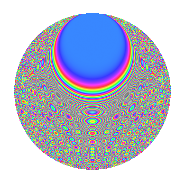# Properties

 Label 672.2.bsLevel 672 Weight 2 Character orbit bs Rep. character $$\chi_{672}(155,\cdot)$$ Character field $$\Q(\zeta_{8})$$ Dimension 384 Newform subspaces 2 Sturm bound 256 Trace bound 4

# Related objects

## Defining parameters

 Level: $$N$$ = $$672 = 2^{5} \cdot 3 \cdot 7$$ Weight: $$k$$ = $$2$$ Character orbit: $$[\chi]$$ = 672.bs (of order $$8$$ and degree $$4$$) Character conductor: $$\operatorname{cond}(\chi)$$ = $$96$$ Character field: $$\Q(\zeta_{8})$$ Newform subspaces: $$2$$ Sturm bound: $$256$$ Trace bound: $$4$$ Distinguishing $$T_p$$: $$5$$

## Dimensions

The following table gives the dimensions of various subspaces of $$M_{2}(672, [\chi])$$.

Total New Old
Modular forms 528 384 144
Cusp forms 496 384 112
Eisenstein series 32 0 32

## Trace form

 $$384q + O(q^{10})$$ $$384q + 16q^{10} + 32q^{16} + 32q^{22} + 48q^{27} - 40q^{30} - 64q^{36} + 48q^{39} - 64q^{46} - 104q^{48} - 64q^{52} - 64q^{55} - 144q^{58} - 56q^{60} + 64q^{61} + 64q^{66} - 64q^{67} - 48q^{70} + 120q^{72} - 112q^{76} + 24q^{78} - 64q^{79} - 112q^{87} + 80q^{88} + 120q^{90} + 96q^{94} + 64q^{96} - 128q^{99} + O(q^{100})$$

## Decomposition of $$S_{2}^{\mathrm{new}}(672, [\chi])$$ into newform subspaces

Label Dim. $$A$$ Field CM Traces $q$-expansion
$$a_2$$ $$a_3$$ $$a_5$$ $$a_7$$
672.2.bs.a $$192$$ $$5.366$$ None $$0$$ $$0$$ $$0$$ $$0$$
672.2.bs.b $$192$$ $$5.366$$ None $$0$$ $$0$$ $$0$$ $$0$$

## Decomposition of $$S_{2}^{\mathrm{old}}(672, [\chi])$$ into lower level spaces

$$S_{2}^{\mathrm{old}}(672, [\chi]) \cong$$ $$S_{2}^{\mathrm{new}}(96, [\chi])$$$$^{\oplus 2}$$

## Hecke Characteristic Polynomials

There are no characteristic polynomials of Hecke operators in the database